SSC JE Civil Past Year Paper Technical - 2017

# SSC JE Civil Past Year Paper Technical - 2017 - Civil Engineering SSC JE (Technical) - Civil Engineering (CE)

CIVIL

Q.1. The largest value of stability number is
(a) 0.261
(b) 0.5
(c) 1.0
(d) 2.0

Ans. (a)
Solution.
Maximum value of stability number is 0.261 and it corresponds to a slope of 90° in purely cohesive soil (Φ = 0). In this case depth factor is equal to 1.

Q.2. Consider the following statements regarding ‘setting of cement’ :
1. Low-heat cement sets faster than OPC.
2. Pinal setting time decides the strength of cement.
3. Initial setting time of Portland Pozzollona is 30 minutes.
4. Air-induced setting is observed when stored under damp conditions. 5. Addition of gypsum retards the setting time.
Which of the above statements are correct?
(a) 1, 2 and 3
(b) 2, 3 and 4
(c) 3, 4 and 5
(d) 2, 3 and 5
Ans. (c)
Solution.
Setting time of low heat cement is same as OPC. Setting times are independent of strength.

Q.3. Extracts from the head-discharge characteristics of two centrifugal pumps are tabulated with respective subscripts 1 and 2; manometric head hm is given in metres; and discharge Q is given in lps:

 Q 12 14 16 18 20 hm1 50.2 50.8 51.3 50 30 hm2 42.4 38.8 35.7 32 25

The pumps are connected in series against a static head of 80 m; the estimate of the total of head losses is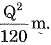What is the delivered discharge?
(a) 15.80 lps
(b) 16.35 lps
(c) 17.35 lps
(d) 17.75 lps

Ans. (a)
Solution.
When pumps are in connected in series, total head is sum of the manometric heads of pumps in series.
H = Hm1 + Hm2
50 + 32 - hL = H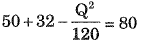[Where Q is in p.s]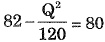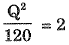Q2 = 120x 2 = 240
Q =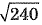≅ 15.8 Ips

Q.4. For sand of uniform spherical particles, the ratio of void ratios in the loosest and the densest states is
(a) 2.6
(b) 3.5
(c) 4.6
(d) 3.0

Ans. (a)
Solution.
Void ratio in loosest state in 0.91 and void ratio in densest is 0.35.

Q.5. Consider the following statements regarding ‘ strength o f cem ent’ :
1. Strength test on cement is made on cubes of cement-sand mix.
2. Water to be used for the paste is 0.25P, where P is the water needed for normal consistency.
3. The normal consistency is determined on Le Chatelier’s apparatus.
4. Cubes are cast in two layers in leak-proof moulds further compacted in each layer by vibration on a machine.
Which of the above statements are correct?
(a) 1 and 2
(b) 2 and 3
(c) 1 and 4
(d) 3 and 4
Ans. (c)
Solution.
Normal consistance is determined on vicat apparatus statement-3 is wrong. Water used for making cement paste is 0.25 p (p is normal consistancy) statement-2 is also wrong.

Q.6. The unit of payment for road side brick edging is in
(a) Cubic meter
(b) Meter
(c) Running meter
(d) Kg
Ans. (c)

Q.7. If damping ratio is 0.10 and damping coefficient is 225 kN sec/m, then critical damping coefficient in kN sec/m will be
(a) 22.5
(b) 225
(c) 2250
(d) 22500
Ans. (c)
Solution.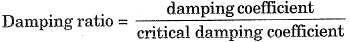Q.8. Which of the following ingredients refer to binding materials of mortar?
1. Cement
2. Lime
3. Sand
4. Ashes

Select the correct answer using the code given below.
(a) 1 and 4
(b) 3 and 4
(c) 1 and 2
(d) 2 and 3
Ans. (c)
Solution.
Sand and ashes can’t be used as a binding material.

Q.9. While estimating for plastering, usually no deduction is made for
(a) Ends of beams
(b) Small openings upto 0.5 sq m
(c) Ends of rafters
(d) All of these
Ans. (d)

Q.10. For a given soil sample,
Cu = coefficient of uniformity
D10 = effective size
D30 = diameter through which 30 per cent of the total soil mass is passing
If Cc is 1.0 and Cu is 4.0, then value of D30/D10 would be
(a) 2.00
(b) 1.75
(c) 1.50
(d) 1.25
Ans. (a)
Solution.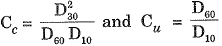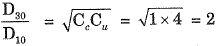Direction (Q.11) : Each of the items consists o f two statements, one labelled as ‘Statement (I)’ and the other as ‘Statement (II)’. You are to examine these two statements and select the answers to these items using the code given below :
Codes:
(a) Both statement (I) and Statement (II) are individually true and statement (II) Is the correct explanation of Statement (I)
(b) Both Statement (I) and Statement (II) are individually true but statement (II) Is not the correct explanation of Statement (I)
(c) Statement (I) is true but Statement (II) is false
(d) Statement (I) is false but Statement (II) is true
Q.11. Statement (I):
Bricks are soaked in water before using in brick masonry for removing dirt and dust.

Statement (II):
Bricks are soaked in water before using in brick masonry so that bricks do not absorb moisture from the bonding cement mortar.

Ans. (d)
Solution.
Bricks are soaked in water not to remove dirt and dust. Dirt and dust can be removed by sprinking with jets of water.
The main purpose of soaking in water is to make it saturated and to avoid absorption of moisture from cement mortar.

Q.12. Ratio of cost of labour to the total cost of the building is
(а) 1 : 10
(b) 1 : 4
(c) 1 : 1

(d) 6 : 10
Ans. (b)

Q.13. The relationship between water content (w%) and number of blows (N) in soils, as obtained from Casagrande’s liquid limit device, is given by
w = 20 - log10 N
The liquid limit of the soil is
(a) 15.6%
(b) 16.6%
(c) 17.6%
(d) 18.6%
Ans. (d)
Solution.
Liquid limit is the water content for 25 number of blows.
∴ LL = 20 - log10 25 = 18.6

Q.14. Direction (Q . 14) : Each o f the items consists o f two statements, one labelled as ‘Statement (I)’ and the other as ‘Statement (II)’. You are to examine these two statements and select the answers to these items using the code given below :
Codes :
(a) Both statement (I) and Statement (II) are individually true and statement (II) is the correct explanation of Statement (I)
(b) Both Statement (I) and Statement (II) are individually true but statement (II) is not the correct explanation of Statement (I)
(c) Statement (I) is true but Statement (II) is false
(d) Statement (I) is false but Statement (II) is true
Q.14. Statement (I):
Preparing mortar by using masonry cement improves workability as well as the finish during plastering.
Statement (II):
Masonry cement is easy to handle

Ans. (a)
Solution.
Masonry cements are workable and give; fine fire finish, therefore these masonry cements are easily handled.

Q.15. Thickness of 25 gauge sheet is
(a)
Less than 1 mm
(b)
1 mm
(c)
Between 1 mm and 1.5 mm
(d)
2 mm
Ans. (a)

Q.16. When the degree of consolidation is 50%, the time factor is about
(a) 0.2
(b) 0.5
(c) 1.0
(d) 2.0
Ans. (a)
Solution.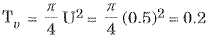Direction (Q.17): Each o f the items consists o f two statements, one labelled as ‘Statement (I)’ and the other as ‘Statement (II)’. You are to examine these two statements and select the answers to these items using the code given below : Codes :
(a) Both statement (I) and Statement (II) are individually true and statement (II) is the correct explanation of Statement (I)
(b) Both Statement (I) and Statement (II) are individually t,rue but statement (II) is not the correct explanation of Statement (I)
(c) Statement (I) is true but Statement (II) is false
(d) Statement (I) is false but Statement (II) is true
Q.17. Statement (I) :
Grading of concrete is based on 28-day strength.
Statement (II):
Concrete does not gain any further strength after 28-day curing.
Ans. (c)
Solution.
Grading of cement concrete is based on 28 day strength. After 28 days there will be slow rate of gain of strength until 1 year.

Q.18. In a detailed estimate the provision for contingencies is, usually
(a) 1 percent
(b) 3 to 5 percent
(c) 10 percent
(d) 12 to 15 percent
Ans. (b)

Q.19. The stress distribution at a depth beneath a loaded area is determined using Newmark’s influence chart which indicate an influence value o f 0.005. The number of the segments covered by the loaded area in the chart is 20 and the intensity of loading on the area is 10 T/m2. The intensity of stress distribution at that depth is
(a) 1 T/m2
(b) 2 T/m2
(c) 5 T/m2
(d) 10 T/m2
Ans. (a)
Solution.
σ= N.IN.q
= 20 x 0.005 x 10
= 1 T/m2

Direction (Q.20) : Each of the items consists of two statements, one labelled as ‘Statement (I)’ and the other as 'Statement (II)’, You are. to examine these two statements and. select the answers to these items using the code given below :
Codes:
(a) Both, statement (I) and Statement (II) are individually true and statement (II) is the correct explanation of Statement (I)
(b) Both Statement (I) and Statement (II) are individually true but statement (II) is not the correct explanation of Statement (I)
(c) Statement (I) is true but Statement (II) is false
(d) Statement (I) is false but Statement (II) is true
Q.20. Statement (I) :

Statement (II) :

Ans. (c)
Solution.
Addition of admixture increases workability but does not increase strength directly. However, increased workability causes good compaction and proper distribution of particles in the mix causes increased strength indirectly.

Q.21. Generally the provision for supervision is
(a) 1 per cent
(b) 2 to 3 percent
(c) 3 to 5 percent
(d) 5 to 10 percent
Ans. (d)

Q.22. If in a flownet diagram, length of the flow line in the last square is 2 m, total head loss is 18 m and the number of potential drops is 12, then value of exit gradient is
(a) 0.33
(b) 0.75
(c) 1.33
(d) 3.00

Ans. (b)
Solution.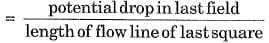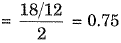Q.23. Which compound of cement is responsible for strength of cement?
(a) Magnesium oxide
(b) Silica
(c) Alumina
(d) Calcium sulphate

Ans. (b)
Solution.
Silica is the compound responsible for primary strength.

Q.24. In specification of earth work in foundation trenches, drains etc. lift ordinarily specified is
(a)
30 m
(b)
5 m
(c)
3 m
(d)
1.5 m
Ans. (d)

Q.25. Under load, the void ratio of a submerged saturated clay decreases from 1.00 to 0.92. What will be the ultimate settlement of the 2 m thick clay due to consolidation?
(a) 20 mm
(b) 40 mm
(c) 80 mm
(d) 160 mm

Ans. (c)
Solution.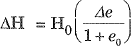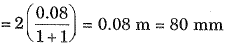Q.26. Which type of t comul v recommended in large mass concrete work;, such as a dam? (а) Ordinary Portland
(b) High Alumina
(c) Low-heat Portland
(d) Portland Pozzollona

Ans. (d)
Solution.
Portland pozzolana cement is in general used for mass construction. It produces low heat and less cracks with higher durability.

Q.27. The useful part of liveable area of a building is called
(а) carpet area
(b) circulation area
(c) horizontal circulation area
(d) plinth area
Ans. (a)

Q.28. The two criteria for the determination of allowable bearing capacity of a foundation are
(а) Tensile failure and compression failure.
(b) Tensile failure and settlement.
(c) Bond failure and shear failure.
(d) Shear failure and settlement.
Ans. (d)

Q.29. Consider the following statements regarding ‘setting o f cement’ :
1. Low-heat cement sets faster than OPC.
2. Final setting time decides the strength of cement.
3. Initial setting time of Portland Pozzollona is 30 minutes.
4. Air-induced setting is observed when stored under damp conditions.
5. Addition of gypsum retards the setting time.
Which of the above statements are correct?
(a) 1, 2 and 3
(b) 2, 3 and 4
(c) 3, 4 and 5
(d) 2, 3 and 5

Ans. (c)
Solution.
Setting time of low heat cement is same as OPC. Setting times are independent of strength.

Q.30. In analysis of rates, contractor profit is taken at the rate of
(a) 1%
(b) 5%
(c) 10%
(d) 20%
Ans. (c)

Q.31. If the effective stress strength parameters of a soil are c'= 10 kPa and o'=30°, the shear strength on a plane within the saturated soil mass at a point where total normal stress is 300 kPa, and pore water pressure is 150 kPa, will be
(a) 90.5 kPa
(b) 96.6 kPa
(c) 101.5 kPa
(d) 105.5 kPa
Ans. (b)
Solution.
S = c + σ' tan Ф
= 10 + (1.50) tan 30 = 96.6 kPa

Q.32. When power shovels are operated under different site conditions (in terms of material handled), what is the correct sequence in the increasing order of the output for the following materials?
1. Well-blasted rock
2. Hard and tough clay
3. Poorly blasted rock
4. Moist loam or sand
Select the correct answer using the code given below:
Codes :
(a) 1-2-3-4
(b) 1-4-3-2
(c) 4-2-3 1
(d) 4-3-1-2

Ans. (c)
Solution.
Power shovels are capable of excavating all classes of earth , except the solid rock with prior loosening.

33. Original cost of property minus depreciation is
(a) Book value
(b) Salvage value
(c) Rateable
(d) Obsolence value
Ans. (a)

34. The Hardy Cross method of hydraulic analysis of pipe networks, besides satisfying the continuity and energy principles, must also satisfy the condition that
(a) Algebraic sum of the head losses around any closed loop is zero
(b) Flow into any junction equals the outflow from it.
(c) Flow in each pipe has head loss according to Darcy-Weisbach or any other pipe head loss equation
(d) Momentum principle is followed.
Ans. (c)
Solution.
Option (a) is due to energy principle and option (b) is due to continuity principle.

35. A double draining clay layer, 6m thick, settles by 30mm in three years under the influence of a certain loads. Its final consolidation settlement has been estimated to be 120mm. If a thin layer of sand having negligible thickness is introduced at a depth of 1.5m below the top surface, then final consolidation settlement of clay layer will be
(a) 60 mm
(b) 120 mm
(c) 240 mm
(d) None of these
Ans. (b)
Solution.
Final settlement will remain same, but rate of settlement will increase.

Q.36. Which is the most common cause of acidity in water ?
(a) Carbon monoxide
(b) Nitrogen
(c) Hydrogen
(d) Carbon dioxide
Ans. (d)
Solution.
CO2 reacts with water to form carbonic acid.

Q.37. If physical quantities involved in a fluid flow phenomenon are discharge Q, diameter D, acceleration due to graity g, dynamic viscosity μ i and density ρ, then number of π arameters needed to express the function F (Q, D, g, m, p) = 0 are
(a) 2
(b) 3
(c) 4
(d) 5
Ans. (a)
Solution.
Since there are five unknown and only three variables (M, L, T), therefore
S - 3 = 2 number of π-parameters are needed

Q.38. In a Mohr’s diagram, a point above Mohr’s envelope indicates
(a) Imaginary condition
(b) Safe condition
(c) Imminent failure condition
(d) Condition of maximum obliquity
Ans. (a)

Q.39. Tool used for quarrying of stones is
(a) Dipper
(b) Spalling hammer
(c) Jumper
(d) Priming needle
Ans. (c)

Q.40. When a particular discharge is flowing in a horizontal pipe, a mercury-water U-tube manometer connected to the entrance and throat of a venturimeter fitted in the pipe recorded a deflection of 25 cm. If same discharge flowed through the same pipe kept at an inclination of 45° to the horizontal, then corresponding deflection by the U-tube manometer will be
(a)  25√2 cm
(b) 25/√2 cm
(c) 22/2 cm
(d) 25 cm
Ans. (d)
Solution.
Deflection in venturimeter for measuring discharge is independent of the angle of pipe.

Q.41. An unit volume of a mass of saturated soil is subjected to horizontal seepage. The saturated unit weight is 22 kN/m3 and the hydraulic gradient is 0.3. The resultant body force on the soil mass is
(a) 1.98 kN
(b) 6.6 kN
(c) 11.49 kN
(d) 22.97 kN
Ans. (b)
Solution.
Seepage force/unit volume = i.yw
= 0.3 x 22
= 6.6 kN

Q.42. If total hardness and alkalinity of a water sample are 200 mg/1 as CaCOg and 260 mg/1 as CaC03 respectively, what are the values of carbonate hardness and non-carbonate hardness ?
(a) 200 mg/1 and zero
(b) Zero and 60 mg/1
(c) Zero and 200 mg/1
(d) 60 mg/1 and zero
Ans. (a)
Solution.
Since alkalinity is more than total hardness so only carbonate hardness is present.
Carbonate hardness = 200 mg/L
Non-carbonate hardness = zero

Q.43. A ship’s model of scale 1 : 100 had a wave resistance of 1 kg at its design speed. The corresponding wave resistance in prototype will be
(a) 100 kg
(b) 10000 kg
(c) 1000000 kg
(d) 1000 kg
Ans. (c)
Solution.
Fr ∝ Lr3
∴ Fp = 1 x (100)3 = 106 kg

Q.44. The maximum average depth due to one day storm over an area of 100 km2 is 10 cm. Depth- Area-Duration (DAD) curves indicate that for the same area of 100 km2 maximum average depth for a 3 hour storm will be
(a) 10 cm
(b) More than 10 cm
(c) Less than 10 cm
(d) None of these
Ans. (b)
Solution.
For a given area, maximum depth increases with duration.

Q.45. In the context of collecting undisturbed soil samples of high quality using a spoon sampler, following statements are made.
I. Area ratio should be less than 10%
II. Clearance ratio should be less than 1%
With reference.to above statements, which of the following applies ?
(а) Both the statements are true
(b) Statement II- is true but I is false
(c) Statement I is true but II is false
(d) Both the statements are false

Ans. (c)
Solution.
For spoon sampler
(i) area ratio should preferably not exeed 10%.
(ii) inside clearance should be between 1 to 3%

Q.46. The increase in metacentric height
1. increases stability
2. decreases stability
3. increases comfort for passengers
4. decreases comfort for passengers
(a) (1) and (3)
(b) (1) and (4)
(c) (2) and (3)
(d) (2) and (4)

Ans. (a)
Solution.
Increase in metacentric height reduces the time period of rolling of the body which is quite uncomfortable for passangers.

Q.47. If 4 hr unit hydrograph of a basin can be approximated as a triangle with a base period 48 h and a peak ordinate of 200 m3/s, then area of basin will be
(a) 1728 km2
(b) 3456 km2
(c) 864 km2
(d) 5184 km2
Ans. (a)
Solution.
Area of basin = 1/2 x 48 x 3600 x 200 = 1728 km2

Q.48. A soil sample is having a specific gravity of 2.60 and a void ratio of 0.78. The water contact in percentage required to fully saturate the soil at that void ratio would be
(a) 10
(b) 30
(c) 50
(d) 70
Ans. (b)
Solution.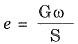S = 1 (saturated soil)

Q.49. Which one of the following velocity fields represents a possible fluid flow ?
(a) u = x; v = y
(b) u = x2; v = y2
(c) u = xy; v = x2y2
(d) u = x; v = - y
Ans. (d)
Solution.
For possible fluid flow,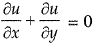Q.50. The direct runoff hydrograph of a basin can be approximated as a triangle with a base period of 80 h and a p eak flow o f 200 m3/s occuring at 16th hour. If area of the basin is 1440 km2, then depth of runoff indicated by the hydrograph will be
(a) 1 cm
(b) 10 cm
(c) 2 cm
(d) 12 cm
Ans. (c)
Solution.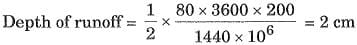Q.51. A loose uniform sand with rounded grains has effective grain size of 0.05 cm. Coefficient of permeability of the sand is
(a) 0.25 cm/sec
(b) 0.50 cm/sec
(c) 1.00 cm/sec
(d) 1.25 cm/sec
Ans. (a)
Solution.
k = 100 D102 cm/sec
= 100 x (0.05)2 cm/sec
= 0.25 cm/sec

Q.52. The critical state of flow through a channel section may be defined as the state of flow at which
(a) specific energy is maximum for a given discharge
(b) specific force is maximum for a given discharge
(c) discharge is maximum for a given specific force
(d) discharge is minimum for a given specific energy.
Ans. (c)
Solution.
Others conditions-for critical flow : Specific energy and force are minimum at a given discharge Froude number = 1

Q.53. When a 2 hour unit hydrograph is available, other unit hydrograph of following duration may be easily prepared except
(a) 4 hours
(b) 5 hours
(c) 1 hour
(d) 8 hours
Ans. (b)
Solution.
Five not being multiple of 2; hence its difficult to prepare a 5 hour unit hydrograph.

Q.54. The amount of irrigation water required to melt the evapotranspiration needs of the crop during its full growth is called
(а) effective rainfall
(b) consumptive
(c) consumptive irrigation requirement
(d) net irrigation requirement
Ans. (c)
Solution.
It is equal to consumptive use-effective rainfall

Q.55. If flow net of a coffer dam foundation had 6 numbers of flow channels and 16 numbers of equipotential drops, with the head of water lost during seepage being 6 m through the foundation having k = 4 x 10-5 m/minute, the seepage loss (in m3/day) per metre length of the dam will be
(a) 2.16 x 10-3
(b) 6.48 x 10-3
(c) 12.96 x 10-2
(d) 25.92 x 10-2
Ans. (c)
Solution.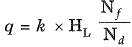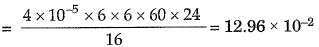Q.56. The equation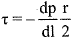for flow through circular tubes, where x is shear stress at distance r from centre, is applicable for
(а) Laminar flow only
(b) Turbulent flow only
(c) Critical flow
(d) Both laminar and turbulent flows.
Ans. (d)
Solution.
Because in deriving the equation, no assumption has been made as nature of flow.

Q.57. A balancing reservoir is one which
(a) Balances the peak and minimum flows
(b) Balances the distribution
(c) Balances the flow rates of supply and demand
(d) Stores water for emergencies

Ans. (c)
Solution.

Balances flow rate of supply and demand.

Q.58. In a saturated soil deposit having a density of 22 kN/m3, the effective normal stress on a horizontal plane at 5 m depth will be
(a) 22 kN/m2
(b) 50 kN/m2
(c) 60 kN/m2
(d) 110 kN/m2

Ans. (c)
Solution.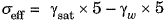= 110 - 50 = 60 kN/m2

Q.59. Which of the following does not fall in the category of displacement method ?
(a) Method of consistent deformation
(b) Equilibrium method
(c) Moment distribution method
(d) Stiffness method
Ans. (a)

Q.60. The unit cohesion of a saturated clay is 1 kg/cm2. The net ultimate bearing capacity of a square footing in this clay will be approximately
(a) 2 t/m2
(b) 10 t/m2
(c) 15 t/m2
(d) 40 t/m2
Ans. (b)
Solution.

qnf = 1.3 c • Nc
= 1.3 x 1 x 5.7 = 10 t/m2

Q.61. Size of a venturimeter is specified by
(a) Pipe diameter
(b) Throat diameter
(c) Angle of diverging section
(d) Both pipe diameter as well as throat diameter.
Ans. (d)

Q.62. A saturated clay layer with single drainage face takes. 4 years to attain 50% degree of consolidation. If clay layer had double drainage, then time to attain 50% degree of consolidation is
(a) 8 year
(b) 4 year
(c) 2 year
(d) 1 year
Ans. (d)
Solution.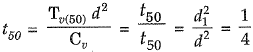Q.63. A fluid is a substance that
(а) Is essentially incompressible
(b) Has a viscosity that always decreases with temperature
(c) Can not remain at rest when subjected to a shearing stress
(d) Can not be subjected to shear forces.
Ans. (c)
Solution.

Options (a) and (b) are true for a liquid but not for gases.
Option (c) is true for ail fluids.

Q.64. Specific yield for an unconfined aquifer is
(a) Greater than porosity
(b) Less than porosity
(c) Equal to porosity
(d) Unrelated to porosity
Ans. (b)
Solution.

Specific yield + Specific retention = Porosity
Thus specific yield, evidently, is less than porosity.

Q.65. Castigliano’s theorem fall under the category of
(a) Displacement method
(b) Equilibrium method
(c) Force method
(d) Stiffness method
Ans. (c)

Q.66. Soil are arranged in face to face orientation. This type of soil structure is
(a) Dispersed
(b) Cohesive matrix
(c) Honey comb
(d) Flocculent
Ans. (a)
Solution.

The soil structure in the form of face to face orientation is called dispersed.

Q.67. In which treatment unit is “Schmutz decke” formed ?
(a) Sedimentation tank
(b) Rapid sand filter
(c) Coagulation tank
(d) Slow sand filter
Ans. (d)
Solution.

Biological layer formed by microbial decomposition of harmless compounds is called schmutzdecke or dirty skin. This helps in absorbing and straining out impurities. This layer is formed in slow sand filter.

Q.68. Under the same conditions, which of the following shapes of water surface will give the highest rate of evaporations ?
(а) Flat water surface
(b) Convex water surface
(c) Concave water surface
(d) Independent of shape of water surface.
Ans. (b)
Solution.

Convex water surface will give the highest rate of evaporation.

Q.69. If in a soil sample piping phenomenon occurs, then what is the most prominent condition to be satisfied?
(a) Soil is fine grained
(b) Hydraulic gradient is close to unity
(c) Void ratio is more than 2.0
(d) Specific gravity of soil solids is more than 2.8

Ans. (b)
Solution.

For a piping phenomenon to occur, hydraulic gradient is 1.

Q.70. Compatibility conditions are essentially required to solve
(a) Substitute frame
(b) Complex truss
(c) Redundant frame
(d) Compound truss
Ans. (c)

Q.71. The maximum bending moment at the left quarter point of a simple beam due to crossing of UDL of length shorter than the span in the direction left to right; would occur after the load had just crossed the section by
(a) One-fourth of its length
(b) Half of its length
(c) Three-fourth of its length
(d) Its full length
Ans. (c)
Solution.

Bending Moment at a section is maximum when load is so placed that section divides the load in same ratio as it divides the span.

Q.72. The correct order of capilliary rise in increasing order in different tyres of soils is
(a) Fine sand, clay, silt, colloids
(b) Silt, fine sands, clay, colloids
(c) Fine sand, clay, colloids, silt
(d) Fine sand, silt, clay, colloids

Ans. (a)
Solution.
Capilliary rise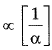Smaller the particle size, more will be the rise.

Q.73. Which of the following is the critical combination of stresses for interior and edge regions in a cement concrete pavements during summer mid day?
(a) Ls - Ws + Fs
(b) Ls + Ws + Fs
(c) Ls + Ws - Fs
(d) Ls - Ws - Fs
where,
Ws = warping stress,
Fg = frictional stress
Ans. (c)
Solution.
Most critical combination of stress is at mid day at edge region in summer season, it is most severe.
Stress (summer) = Load stress + Warping stress - Frictional stress

Q.74. Moment distribution method is best suited for
(a) Indeterminate pin jointed truss
(b) Rigid frames
(c) Space frames
(d) Trussed beam
Ans. (b)

Q.75. Due to some point load anywhere on a fixed beam, the maximum free bending moment is M, The sum of fixed end moment is
(a) M
(b) 1.5 M
(c) 2.0 M
(d) 3.0 M

Ans. (a)
Solution.
Maximum free BM,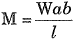Sum of fixed end moments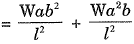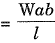Q.76. Taylors stability number curves are used for the analysis of stability of slopes. The angle of shearing resistance used in the chart is the
(a) Mobilised angle
(b) Weighted angle
(c) Effective angle
(d) Apparent angle

Ans. (a)
Solution.

For an analysis of stability of slopes by Taylors method, angle of shearing resistance used in chart is the mobilised angle.

Q.77. The critical condition ofloading for combination of stresses in cement concrete roads for corner region is
(a) Load stress + frictional stress
(b) Load stress + warping stress
(c) Load stress + warping stress + frictional stress
(d) Load stress + warping stress - frictional stress
Ans. (c)
Solution.

C.C. = Load stress + Warping stress at corner

Q.78. The horizontal thrust due to rise in temperature in a semi-circular two hinged arch of radius R is proportional to
(a) R
(b) R2
(c) 1/R
(d) 1/R2
Ans. (d)
Solution.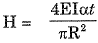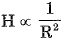Q.79. If azimuths of the two tangents to a circular curve of radius 100 m are due north and due east, then area bounded by the two tangents and the circular curve will be
(a) 7857 sqm
(b) 5000 sqm
(c) 3143 sqm
(d) 2143 sqm
Ans. (d)
Solution.

Bounded area =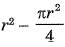10000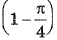= 2146 sq m .

Q.80. If unconfined compressive strength of a clay in undisturbed and remoulded state are 4.5 kg/cm2 and 1.5 kg/cm2 respectively, then sensitivity of clay will be
(a) 2.0
(b) 3.0
(c) 0.5
(d) 5.0
Ans. (b)
Solution.

Sensitivity is the ratio of undisturbed shear stress to shear stress of remoulded soil sample stress.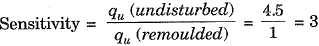Q.81. Which of the following figure shows the relationship between traffic speed and traffic density?
(a)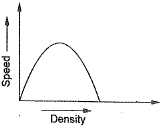(b)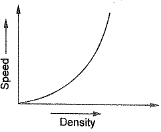(c)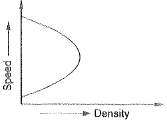(d)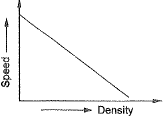Ans. (d)
Solution.

It is seen as velocity increases distance between vehicles increases and density decreases.

Q.82. If parallax difference between top and bottom of a tree is measured as 1.32 mm on a stereopair of photos taken at 3000 m above ground and average photo base is 66 mm, then height of the tree will be
(a) 45.49 m
(b) 60.00 m
(c) 23.51m
(d) 39.50 m

Ans. (b)
Solution.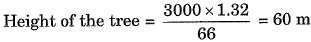Q.83. Negative skin friction on a pile under vertical compressive load acts
(a) Downward and increases the load carrying capacity of the pile.
(b) Downward and reduces the load carrying capacity of the pile.
(c) Upwards and increases the load carrying capacity of the pile.
(d) Downwards and maintains the same load carrying capacity of the pile.
Ans. (b)
Solution.

Negative skin friction on a body acts downward, thus increasing the load resulting in reduction of load carrying capacity of pile.

Q.84. If staff intercept on a staff located at 100 m from the level for five division deviation of the bubble is 0.050 m and if length of one division of the bubble is 2 mm, then radius of curvature of the bubble tube is
(a) 2.02 m
(b) 2.20 m
(c) 20.00 m
(d) 20.20 m
Ans. (c)
Solution.

Radius of curvature of bubble tube is given by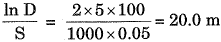Q.85. An index map used in highway project shows
(а) Proposed and existing roads and important places to be connected
(b) General topography of the area
(c) Details of various alternate alignments
(d) General details of existing structures like buildings, wells etc.

Ans. (b)
Solution.

An index map used in highway project shows General Topography

Q.86. Given that a single degree of freedom, k= stiffness co-efficient; m-mass of machine and foundation, then critical damping is best defined by the expression
(a) 2πK√m
(b) 4.2 √km
(c)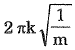(d)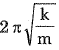Ans. (b)
Solution.

Critical damping is directly proportional to square root of mass and stiffness coefficient in a single degree of freedom system.

Q.87. The Local Sidereal Time (LST) is always
(a) Ahead of the Local Mean Time (LMT) by about 2 hours per month
(b) Behind LMT by about 2 hours per month
(d) Equal to LMT
Ans. (b)
Solution.

One sidereal day,
mean time- 1 solar day - 3 min. 55.91sec.

Q.88. Consider the following statements :
1. Brickwork will have high water tightness.
2. Brickwork is preferred for monument structures.
3. Bricks resist fire better than stones.
4. Bricks of good quality shall have thin mortar bonds.

Which of the above statements are correct?
(a) 1 and 2
(b) 3 and 4
(c) 2 and 3
(d) 1 and 4

Ans. (b)
Solution.

Brick work may not give complete water tightness and ingeneral stonework is preferred for monumental structures.

Q.89. The shift of transition curve of radius 300 m and length 48 m is
(a) 0.32 m
(b) 0.42 m
(c) 0.52 m
(d) 0.62 m

Ans. (a)
Solution.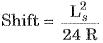Q.90. Pile caps are used on a group of piles to
(a) Increase the load bearing capacity of each pile.
(b) Protect the piles from lateral displacement.
(c) Protect in case of offshore structure.

Ans. (d)
Solution.

Pile caps acts as a joints which connects each pile to one another and this ensures spread of horizontal and vertical loads to all the piles.

Q.91. Consider the following statements :
1. Noise pollution can be reduced using doubleglass window panes.
2. Glass absorbs the noise.
3. The air trapped in the double-glass system acts as an insulator and reduces the noise.
4. The noise totally reflects back due to the two layers of glass.

Which of these statements are correct?
(a) 1, 2, 3 and 4
(b) 1, 2 and 3 only
(c) 1 and 3 only
(d) 2 and 4 only

Ans. (c)
Solution.

Glass not absorbs the noise. And the noise is not otally reflects back. Glass acts as insulator and reduce the noise.

Q.92. What is the minimum sight distance required to avoid a head-on collision of two cars approaching from the opposite directions at 90 and 60 kmph? It is given that reaction time in 2.5 second, coefficient of friction is 0.7 and a break efficiency is 50 per cent in either case.
(a) 153.6 m
(b) 82.2 m
(c) 71.4 m
(d) 235.8 m
Ans. (d)
Solution.

Sight distance to avoid head on collision
= SD1 + SD2

Q.93. Noise pollution in a road-side building can be reduced by
(a)
Providing a ditch around the building and filling it with water
(b)
Providing a steel mesh around the building
(c)
Providing a thick bush around the building
(d)
Planting tall trees around the building and fencing them with barbed wires
Ans. (c)

Q.94. The collapsible soil is associated with
(a) Dune sands
(b) Laterite soils
(c) Loess
(d) Black cotton soils

Ans. (c)
Solution.

Loess is collapsible soil, while black cotton soil is expansive soil.

Q. Direction: The following item consist of two statements, one labelled as the Assertion (A)’ and the other as Reason (R)’. You are to examine these two statements carefully and select the answers to these items using the codes given below :
Codes:
(a) Both A and R are individually true and R is the correct explanation of A
(b) Both A and R are individually true but R is not the correct explanation of A
(c) A is true but R is false
(d) A is false but R is true
Q.95. Assertion (A):
At the standard temperature, the kinematic viscosity of air is greater than that of water at the same temperature.
Reason (R) :
The dynamic viscosity of air at standard temperature is lower than that of water at the same temperature.
Ans. (b)
Solution.

Kinematic viscosity of air is greater because density of air is very small as compared to water.

Q.96. What type of noise can be abated by providing lining on walls and ceiling with sound absorbing materials?
(a)
Source noise
(b)
Reflection noise
(c)
Structural noise
(d)
Direct air-borne noise
Ans. (b)

Q.97. The settlement analysis for a clay layer draining from top and bottom shows a settlement of 2.5 cm in 4 years and an ultimate settlement of 10 cm. However detailed subsurface investigation reveals that there is no drainage at the bottom. The ultimate settlement in this condition will be
(a) 2.5 cm
(b) 5 cm
(c) 10 cm
(d) 20 cm
Ans. (c)
Solution.

Ultimate settlement does not change.

Q.98. Which. of the following pairs are correctly matched ?
1. Reverberation - Time require time to reduce noise by 60 dB
2. NIPTS - Responsible for permanent hearing loss
3. Sound foci - Formed when sound waves are reflected from convex surface
4. TTS - Responsible for temporary hearing loss
Select the correct given below: answer using the codes
(a) 2, 3 and 4
(b) 1,3 and 4
(c) 1,2 and 4
(d) 1,2 and 3
Ans. (a)

Direction(Q.99) : Each o f the items consists o f two statements, one labelled as the ‘Statement (I)’and the other as ‘Statement (II)’. You are to examine these two statements carefully and select the answers to these items using the codes given below
Q.99. Statement (I):
‘Environment’ includes abiotic and biotic parameters.
Statement (II):
Abiotic parameters include algae, bacteria, animals; and biotic parameters are air, water and soil.
Ans. (c)

Q.100. A soil sample tested in a triaxial compression apparatus failed when total maximum and minimum principal stresses were 100 kPa and 40 kPa, respectively. The pore pressure measured at failure was 10 kPa. The effective principal stress ratio at failure is
(a) 2.5
(b) 3.0
(c) 2.75
(d) 2.0
Ans. (b)
Solution.

Effective principal stress ratio at failure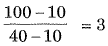The document SSC JE Civil Past Year Paper Technical - 2017 | Civil Engineering SSC JE (Technical) - Civil Engineering (CE) is a part of the Civil Engineering (CE) Course Civil Engineering SSC JE (Technical).
All you need of Civil Engineering (CE) at this link: Civil Engineering (CE)

## FAQs on SSC JE Civil Past Year Paper Technical - 2017 - Civil Engineering SSC JE (Technical) - Civil Engineering (CE)

 1. What is the SSC JE Civil Exam?Ans. The SSC JE Civil Exam is a competitive examination conducted by the Staff Selection Commission (SSC) for the recruitment of Junior Engineers (JE) in the civil engineering field.
 2. What is the eligibility criteria for the SSC JE Civil Exam?Ans. To be eligible for the SSC JE Civil Exam, candidates must have a degree or diploma in civil engineering from a recognized university or institute. The age limit for the exam is between 18 to 32 years, although age relaxations are provided for reserved category candidates.
 3. What is the exam pattern for the SSC JE Civil Exam?Ans. The SSC JE Civil Exam consists of two papers - Paper 1 and Paper 2. Paper 1 is an objective type exam with questions on general intelligence and reasoning, general awareness, and civil engineering. Paper 2 is a descriptive type exam that tests the candidate's knowledge in civil engineering.
 4. How can I prepare for the SSC JE Civil Exam?Ans. To prepare for the SSC JE Civil Exam, candidates should start by understanding the exam pattern and syllabus. They can then create a study plan, gather relevant study materials, and practice previous year question papers and mock tests. It is also recommended to revise regularly and stay updated with current affairs related to civil engineering.
 5. What are the job prospects after clearing the SSC JE Civil Exam?Ans. After clearing the SSC JE Civil Exam, candidates are eligible for various job opportunities in government departments and organizations. They can work as Junior Engineers in departments like Central Public Works Department (CPWD), Military Engineering Service (MES), Central Water Commission (CWC), and many more.

## Civil Engineering SSC JE (Technical)

2 videos|122 docs|55 tests

## Civil Engineering SSC JE (Technical)

2 videos|122 docs|55 tests
Signup to see your scores go up within 7 days! Learn & Practice with 1000+ FREE Notes, Videos & Tests.
10M+ students study on EduRev
Track your progress, build streaks, highlight & save important lessons and more!(Scan QR code)
Related Searches

,

,

,

,

,

,

,

,

,

,

,

,

,

,

,

,

,

,

,

,

,

;